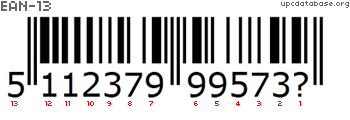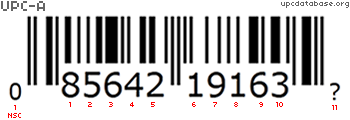### Check Digit Calculators

A check digit, by definition, is a form of redundancy check used for error detection, the decimal equivalent of a binary checksum. It consists of a single digit computed from the other digits in the message. -Wikipedia

The check digit calculation is the computers own way of knowing if an item got scanned correctly. When a barcode is scanned, the group of digits are sent to the computer or register which instantly calculates the check digit based on the calculations found below. If the check digit does not match, the computer informs the teller to either rescan the item or to enter the digits manually.

There are a number of reasons why a check digit check would fail. The item could have been scanned too quickly, their may be some dirt between the lines, or an items packaging may just be folded or crinkled.

Check Digit Calculator
Unknown

This check digit calculator currently works on EAN, ISBN, AND UPC numbers.

### How does it work?

EAN-13 Check Digit CalculationsStep 1 Add the values for all the even-numbered positions.
1+2+7+9+5+3=27
Step 2 Multiply the above answer by 3.
27*3=81
Step 3 Add the values for all the odd-numbered positions.
5+1+3+9+9+7=34
Step 4 Add the answers from step 2 and 3 together.
81+34=115
Step 5 If the answer from step 4 ends in a "0", then the check digit is "0". Otherwise, subtract the number from step 4 from the next highest multiple of 10 to get the check digit.
120-115=5
EAN-13 Check Digit CalculationsStep 1 Add the values for all the odd-numbered positions.
0+5+4+1+1+3=14
Step 2 Multiply the above answer by 3.
14*3=42
Step 3 Add the values for all the odd-numbered positions.
8+6+2+9+6=31
Step 4 Add the answers from step 2 and 3 together.
42+31=73
Step 5 If the answer from step 4 ends in a "0", then the check digit is "0". Otherwise, subtract the number from step 4 from the next highest multiple of 10 to get the check digit.
80-73=7